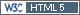## Educate the IEC and regulators [Power / Sample Size]

Hi Yura,

» You are considering the power after the study at n = 28 (which were calculated before the study: GMR=0.95, CV=0.25, β=0.80). The question is whether it is possible to carry out a study at n = 50 and will this be forced bioequivalence?

As I wrote above the IEC and the authority should judge this before the study is done. I agree that in many cases the statistical knowledge of IECs is limited. However, once the protocol was approved by both, I don’t see a reason to talk about “forced BE” any more.

BTW, I don’t see a problem if a study is designed for 90% power (80% is not carved in stone). Let’s assume a dropout rate of 15% and we will already end up with 46 subjects:

```library(PowerTOST) CV     <- 0.25 # CV-intra theta0 <- 0.95 # T/R-ratio target <- 0.90 # desired (target) power dor    <- 15   # expected dropout rate in percent n      <- sampleN.TOST(CV=CV, theta0=theta0, targetpower=target,                        print=FALSE)[["Sample size"]] ceiling(n/(1-dor/100)/2)*2 # round up to next even```

Considering your example and assuming that the GMR and CV turn out exactly as assumed, no dropouts (n=50): The 90% CI will be 87.47–103.18%. Fine with me. Not even a significant difference (100% included). If the drop­out-rate is as expected (n=38) the 90% CI will be 86.36–104.51%. If the assessor is not happy with that, he should have a chat with his colleague who approved the protocol and enlighten him about potential “over-powering” in study planing.
According to all guidelines (CI within the acceptance range) I can’t imagine a justification to reject the study. If the study is not accepted only due to the high sample size in the EEA the applicant might go for a referral (with extremely high chances of success) and in the USA the FDA will be sued right away.Cheers,
Helmut SchützThe quality of responses received is directly proportional to the quality of the question asked. ☼
Science QuotesIng. Helmut Schütz